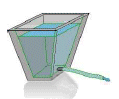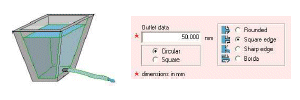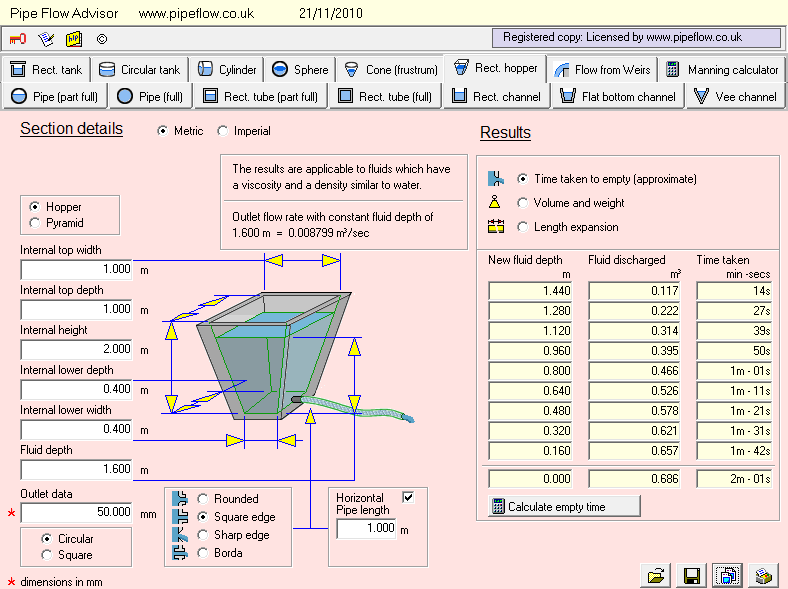# Tank Emptying Time CalculatorThe Pipe Flow Advisor software can calculate tank weight, tank capacity, tank expansion due to temperature change, volume for a given height of fluid, discharge flow rate, and the time taken for the tank to empty with flow under gravity.

## Tank Outlet Type / Orifice Type

The tank's discharge flow rate through the exit orifice is dependent on a number of factors:

• The Discharge Coefficient (which varies with different types of outlet fitting).
• The Cross Sectional Area of the orifice.
• The Head of Liquid above the orifice outlet point.

The software can calculate flow rate results for a number of different outlet types:• Rounded edge outlet - discharge coefficient 0.98
• Square edge outlet - discharge coefficient 0.80
• Sharp edge outlet - discharge coefficient 0.61
• Borda outlet (projecting inside) - discharge coefficient 0.51

## Tank Emptying Time CalculatorThe tank discharge flow rate through its exit orifice is calculated as follows:

Flow rate =
orifice discharge coefficient x
orifice cross-sectional area x
square root (2gH)

where g = acceleration due to gravity
where H = head of fluid above the outlet

As the tank empties, the remaining fluid head inside the tank reduces and therefore the pressure at the exit point reduces, hence the discharge flow rate through the exit orifice also reduces over-time.

### Fluid Surface Area

If the fluid surface area changes as the height of fluid in the tank changes then as flow exits the tank, the rate of change in the height of fluid head will not be constant. Put another way, if the shape of the tank is not consistent over the full height of the tank then when the tank is emptying the height of fluid in the tank will not decrease at a constant rate in relation to the volume of flow that exist the tank

An example using a certain size of hopper tank shows that the time to taken for the fluid level to first reduce by 300 mm (12 inches) is initially 3 min 37 secs, where as the final 300 mm of fluid takes only 1 min 33 secs to empty from the tank.

### Effect of Discharge Pipe on Flow Rate and Empty Time

If a discharge pipe is connected to the tank to deliver the flow to some other point then friction losses in the discharge pipe may affect and restrict the flow rate from the tank. is delivered to

For a particular example, when a 4 metre (13 ft) length of discharge pipe was used, the tank emptying time increased by almost 8 minutes due to a reduced discharge flow rate.

Next: Tank Volume and Weight Calculator

Secure Online PaymentsPipe Flow Software: Piping design, Pressure drop calculator, Flow rate calculator, Pump head calculations, Pump selection software.  Copyright © Pipe Flow Software 1997-2023